# Triangle: Basics and definition

Do you ever thought that,

• How the distance of sun and different planet is measured from earth without having measuring scale of that length?
• by what measure height of mountains calculated?
• How satellite is fixed at some exact position without having distance measurement?

The answer to all these questions is given by scientists by the use of triangle for different measurement and calculation.

In Mathematics geometry plays an important role especially triangle as every universal scientific measurement is done by the use of triangles.

## Definition:

A 2-dimensional plane figure or polygon having sides, vertex and angles all three in number is said to be a triangle. We are much aware of a triangle, its type and some properties related to it.

## There are 3 types of triangle mainly on basis of sides:

1. Equilateral Triangle: In a triangle if all the three sides and angles are equal and angle equal to 60⁰.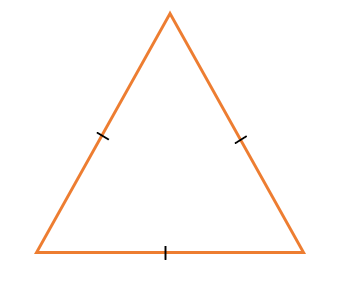2. Isosceles Triangle: In a triangle if two sides are equal and angle adjacent to it are also equal then the triangle is said to be isosceles triangle.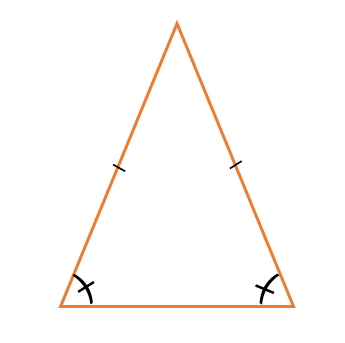3. Scalene triangle: When a triangle has none of the sides, as well as angles, equal then the triangle is said to be scalene triangle.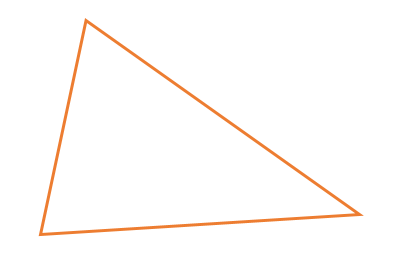### There are 3 types of triangle mainly on basis of internal angle:

1. Right triangle: A triangle in which one of the internal angle measures 90⁰is said to be right triangle.
• In this triangle, the largest of the three sides which lies opposite to the right angle is called hypotenuse.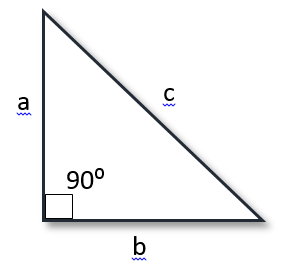Pythagorean Theorem: The sum of squares of the adjacent sides attached to the right angle is equal to the square of hypotenuse.
i.e. a2 + b2 = c2

1. Acute Triangle: When internal angle of a triangle has measure of less than 90⁰. It is said to be acute triangle.
2. Obtuse Triangle: When an internal angle of a triangle has measure of more than 90⁰. It is said to be obtuse triangle.

## Congruence of triangles:

Two triangles are said to be congruent if and only if the first triangle exactly fits the other triangle.

Or, simply we can say that shape and size of one triangle should exactly match to other triangle. Then only they will be congruent.

### There are different theorem to compare the triangles whether they are congruent or not:

1. SAS congruence rule:

By this rule if 2 sides of a triangle and the angle included between it is equal to the other triangle. Thus they are congruent.1. ASA congruence rule:

By this rule if 2 angles of a triangle and the side included between it is equal to the other triangle. Thus they are congruent.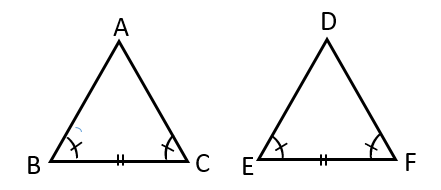1. SSS congruence rule:

By this rule if all sides of a triangle is equal to the other triangle then they are congruent.1. RHS congruence rule:

If two right triangles are compared and hypotenuse and any one side of the triangle is equal to other triangle. Thus the triangles are said to be congruent according to RHS rule.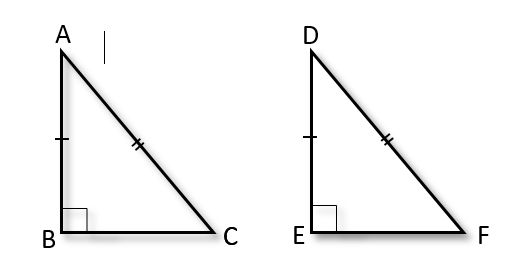## Similarity of Triangles:

Two triangles are said to be similar if measure of every angle of the first triangle is equal to the other triangle and also if the corresponding sides of both triangles are in the same ratio.

Remember: The ratio of any two corresponding sides in two equiangular triangles is always the same.

### Rules for similarity of triangles:

1. AAA similarity rule:

If all the angles of one triangle is equal to the other triangle then it is said to be similar by AAA rule. AAA stands for (Angle – Angle – Angle)

1. SSS similarity rule:

If in two triangles, sides of one triangle are proportional to (i.e., in the same ratio of ) the sides of the other triangle, then their corresponding angles are also equal and hence the two triangles are similiar.

1. SAS similarity rule:

If any one angle of a triangle is equal to angle of the other triangle and the sides attached to the angles are proportional, then the two triangles are similar.

Remember: “The ratio of the areas of two similar triangles is equal to the square of the ratio of their corresponding sides”.

## Terminology Related to triangles:

1. Circumcenter: The point in the plane of triangle where Perpendicular bisectors of all the three sides meet is circumcenter of that triangle. Denoted by “O” generally.
2. Orthocenter: The point at which the altitude of all the three sides meet is called orthocenter. Generally denoted by “H”.
3. Incenter: The point at which the angle bisector of all the three angles meet is said to be incenter of that triangle. Generally denoted by “I”.
4. Centroid: The point at which the median from all the three sides meet is said to be centroid of that triangle. Generally denoted by “G”.

## Area of triangle:

For triangles generally, we use Area = 1/2 b x h